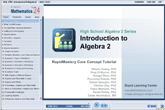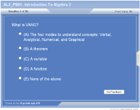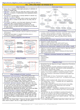How to Learn in 24 Hours?

 Need Help? M-F: 9am-5pm(PST): Toll-Free: (877) RAPID-10 or 1-877-727-4310 24/7 Online Technical Support: The Rapid Support Center vip@rapidlearningcenter.com Secure Online Order:Need Proof? Testimonials by Our Users

 Rapid Learning Courses: MCAT in 24 Hours (2021-22) USMLE in 24 Hours (Boards) Chemistry in 24 Hours Biology in 24 Hours Physics in 24 Hours Mathematics in 24 Hours Psychology in 24 Hours SAT in 24 Hours ACT in 24 Hours AP in 24 Hours CLEP in 24 Hours DAT in 24 Hours (Dental) OAT in 24 Hours (Optometry) PCAT in 24 Hours (Pharmacy) Nursing Entrance Exams Certification in 24 Hours eBook - Survival Kits Audiobooks (MP3)

 Tell-A-Friend: Have friends taking science and math courses too? Tell them about our rapid learning system.Home »  Mathematics »  High School Algebra 2

Introduction to Algebra 2

 Topic Review on "Title": Algebra 2: Algebra 2 is a branch of mathematics that substitutes letters for numbers and uses simplification techniques to solve equations. Algebraic equations: A scale, what is done on one side of the scale with a number is also done to the other side of the scale. Variables: Variables are symbols that are usually defined to denote any member of a set of objects. Functions: Functions are relations defined in such a way that for each element of a set there is a unique element of another set that is associated it with. VANG: VANG stands for Verbal Analytical/Algebraic Numerical Graphical

Rapid Study Kit for "Title":
 Flash Movie Flash Game Flash Card Core Concept Tutorial Problem Solving Drill Review Cheat Sheet"Title" Tutorial Summary : Algebra 2 is a course that involves relations and its use. Algebra 2 along with an Algebra I course provides a solid foundation to higher mathematics course such as Pre-Calculus and Calculus. To be proficient in mathematics, we highly recommend you to examine the examples and properties discussed in this course and ponder any of the subtleties encountered in any mathematics problems.    This tutorial gives an overview of what to look forward to in this algebra 2 course. This introductory tutorial summarized the most important principles encountered in an Algebra 2 course. It is a strong tool to use for anyone who is unfamiliar or is learning algebra 2 for the very first time. The meaning of VANG is discussed in this tutorial.

 Tutorial Features: Specific Tutorial Features: • Definition of algebra 2 and its relationship with other mathematics courses is discussed in this tutorial. • The use of relations and its definition is shown here with the use of step by step examples. Series Features: • Concept map showing inter-connections of concepts introduced. • Definition slides introduce terms as they are needed. • Examples given throughout to illustrate how the concepts apply. • A concise summary is given at the conclusion of the tutorial.

 "Title" Topic List: Getting to Know Algebra 2 What is Algebra 2? Algebra 2 and relations The construction of relations and examplesAlgebra 2 and Other Mathematics Courses Differences and similarities between algebra 2 and other mathematics coursesAlgebra 2 Tips VANG

See all 24 lessons in High School Algebra 2, including concept tutorials, problem drills and cheat sheets:
Teach Yourself High School Algebra 2 Visually in 24 Hours